# Deep Copy and Shallow Copy in C++

## Deep Copy and Shallow Copy in C++ with Examples:

In this article, I am going to discuss Deep Copy and Shallow Copy in C++ with Examples. We have seen different Types of Constructors in our previous article and one of the constructors is the copy constructor that we have already discussed in our previous article.

##### Deep Copy and Shallow Copy in C++

Creating a copy of an object by copying data of all member variables as it is, is called shallow copy while creating an object by copying data of another object along with the values of memory resources that reside outside the object but handled by that object, is called deep copy.

In general, creating a copy of an object means creating an exact replica of the object having the same literal value, data type, and resources.

Depending upon the resources like dynamic memory held by the object, either we need to perform Shallow Copy or Deep Copy in order to create a replica of the object. In general, if the variables of an object have been dynamically allocated then it is required to do a Deep Copy in order to create a copy of the object.

In shallow copy, an object is created by simply copying the data of all variables of the original object. This works well if none of the variables of the object are defined in the heap section of memory. If some variables are dynamically allocated memory from the heap section, then copied object variable will also reference the same memory location.

This will create ambiguity and run-time errors dangling pointer. Since both objects will reference the same memory location, then change made by one will reflect those change in another object as well. Since we wanted to create a replica of the object, this purpose will not be filled by Shallow copy.

##### Example to Understand Shallow Copy and Deep Copy Constructors in C++:

Now let us see the problem with Shallow copy and then we will understand the need and use of a deep copy constructor in C++. Please have a look at the below example.

```class Test
{
int a;
int *p;
Test (int x)
{
a = x;
p = new int[a];
}
Test (Test &t)
{
a = t.a;
p = t.p;
}
};
```

Here we have a class called Test with two constructors i.e. with one parameterized constructor and one copy constructor. We already discussed that we can define more than one constructor in a class i.e. constructor overloading. Next, we have the main function as,

int main(){
Test t (3);
}

Here we have created an object “t” of class “Test” with the value of 3. Now let us study the class and see what will be created.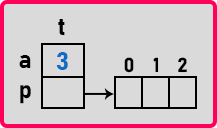Here, the first constructor i.e. Test (int x) will be called as we are passing an integer value as an argument. Inside the constructor, a will assign to 3 and p will point to the address of 3 blocks of memory as we have created an array inside the heap of size a i.e. 3. Next, we will create another object that is t2 and we will pass t as an argument as follows.

int main(){
Test t (5);
Test t2 (t);
}

So, which constructor we are calling? As we are passing ‘t’ as a parameter, so we are calling the copy constructor. Now for object ‘t2’, again memory is allocated for data members a and p.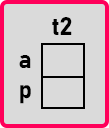Now let us see what the copy constructor is doing.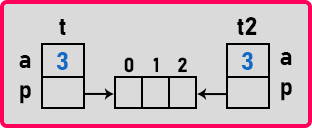Here t2.a is assigned to t.a. Which t? Object ‘t’ that we have created first. So t2.a is assigned to 3. Then t2.p will assign to t.p that is t2.p and t.p will point to the same memory address. This is wrong. This t2 object should have its own array of the same size.

Suppose this ‘t’ is mine and I am having my own array and you wanted the copy of this. So, you created an object like mine but you’re not having a new array. You are pointing to my array. Only I help you with copying. You should create your own array. Why you are pointing to my array? For you, a new array should be created.

So, the problem with the copy constructor is that if there is a dynamic memory allocation (memory allocation in the heap section) done by an object then the copy constructor will not create a new memory for it. It will point to the same memory. So, you have to be careful with this type of thing. So, what we are supposed to do here that we should modify the copy constructor as

Test(Test &t){
a = t.a;
p = new int [a];
}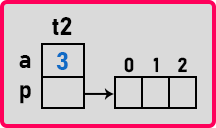So here everything will be copied and a new array will be created by the copy constructor. So, whenever you required a copy constructor just observe and find out whether you need a shallow copy constructor or a deep copy constructor.

And one more thing, if suppose already ‘t’ is having some elements in the array then also you should copy them. Everything we should take care of. Not just creating an array, if it is having the values copy the values also. So now let us write the complete program in C++ language.

##### Example to Understand Shallow Copy Constructor in C++:
```#include <iostream>
using namespace std;

class Test
{
public:
int a;
int *p;

Test (int x)
{
a = x;
p = new int[a];
}
Test (Test & t)
{
a = t.a;
p = t.p;
}
};

int main()
{
Test t (5);
t.p = 1;
Test t2 (t);
cout << "t: " << t.a << " " << t.p << endl;
cout << "t2: " << t2.a << " " << t2.p << endl;
}
```
###### Output: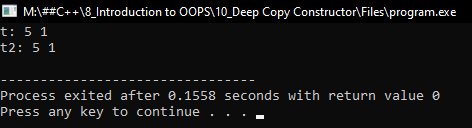##### Example to Understand Deep Copy Constructor in C++:
```#include <iostream>
using namespace std;

class Test
{
public:
int a;
int *p;

Test (int x)
{
a = x;
p = new int[a];
}
Test (Test & t)
{
a = t.a;
p = new int[a];
if (p)
{
for (int i = 0; i < a; i++)
{
p[i] = t.p[i];
}
}
}
};

int main()
{
Test t (5);
t.p = 1;
Test t2 (t);
cout << "t: " << t.a << " " << t.p << endl;
cout << "t2: " << t2.a << " " << t2.p << endl;
}
```
###### Output: